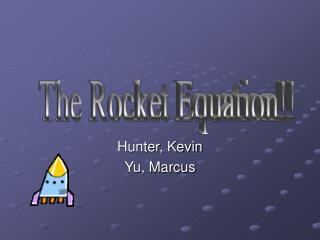DownloadDownload PresentationHunter, Kevin Yu, Marcus

Hunter, Kevin Yu, Marcus

Télécharger la présentationHunter, Kevin Yu, Marcus

- - - - - - - - - - - - - - - - - - - - - - - - - - - E N D - - - - - - - - - - - - - - - - - - - - - - - - - - -
Presentation Transcript

1. The Rocket Equation!! Hunter, Kevin Yu, Marcus

2. These Next Few Steps • Using the Newton Law of motion and some outside research, we will derive the basic equation that describe the rocket launching motion. • The differential equation will give us the rate equation modeling the rocket.

3. Newton’s 1st Law of Motion • “A body in uniform motion remains in uniform motion and a body at rest remains at rest, unless acted on by a non-zero net force” • A rocket will stay at rest unless acted upon. • If a rocket’s thrust can overcome Its weight and gravity, there will be liftoff.

4. Newton’s 2nd Law of Motion • “The rate at which a body’s momentum changes is equal to the net force action on the body” • Fnet = dP/dt • Force = change of momentum over change in time • Force = change of mass*velocity over change in time • F= mass*acceleration (only if mass is constant) • Because rockets burn fuel up, mass Isn’t constant. We need another way to describe momentum that takes in the factor of changing mass!

5. Newton’s 3rd Law of Motion • “If object A exerts a force on object B, then object B exerts an opposite force of equal magnitude” • Thrust is what makes these rockets fly! • When the thrust of the rocket is greater than the outside force such as gravity, drag, and the weight of the rocket, then the rocket will be able to travel in the positive direction, while the exhaust of the rocket will travel in the negative opposite direction.

6. Theoretical approach to finding Thrust • For liftoff: • Thrust > weight * gravity * drag… • Drag = Cd(pV2/2)A • A = area, Cd = Drag Coefficient • P = gas density

7. Thrust equation: • Thrust = F = MVe+ (Pe-Po) Ae • Where Ve = Velocity of Exhaust • Po = Atmosphere Pressure • Pe = Exhaust Pressure • M = mass flow rate

8. Lift off equation • Ms = Mass of Solids (i.e.: Rocket, payload, navigation, etc.) • Ml = Mass of Liquid Rocket Fuel • MT = Mass of Rocket System • <Ms+Ml = MT> • v= velocity of rocket Based off of Newton’s 2nd Law of Motion: • Momentum = mass*velocity (only when mass is constant) • When the rockets fire, Ml changes. So… • Momentum P = (Ms + ΔMl)v • …when the rockets fire, the exhaust comes out at some speed ve relative to the speed of the rocket. Therefore the momentum of the exhaust is • ΔMl(v-ve)

9. Lift off equation: • The Rocket’s mass will eventually drop to Ms. At the same time, its speed increases a small amount (because of a lighter load). • Ms (v + Δv) • But with no external force, the rocket system is at a stand still. So we must sum the two parts together to form the initial momentum. • P = (Ms + ΔMl)v • (Ms + ΔMl)v = Ms (v + Δv) + ΔMl(v-ve) • By multiplying and simplifying: • F = Ms Δv - Δ Ml Ve • F is a force outside of the rocket system (i.e.: gravity) • Shown Differentially • F= Ms(dv/dt) – vex(dMl/dt)

10. Lift off equation: • F= Ms(dv/dt) – vex(dMl/dt) • vex(dMl/dt) = Thrust • So… • F= Ms(dv/dt) - MVe+ (Pe-Po) Ae • Our rate equation for momentum of the Rocket • dP/dt = Ms(dv/dt) - [MVe+ (Pe-Po) Ae]

11. Rocket equation’s application! • Space Shuttle Columbia’s engine ejects mass at a rate of 30 kg/s with an exhaust velocity of 3,100 m/s. The pressure at the nozzle exit is 5 kPa and the exit area is 0.7 m2. The mass of the shuttle solids (not including fuel) is about 500,000 kilograms. Find the acceleration of the rocket.

12. Variables • Given: • M = 30 kg/s • Ve = 3,100 m/s • Ae = 0.7 m2 • Po = 0 • Pe = 5,000 N/m2 • Ms = 500,000kg

13. Calculation • Using the rate equation : • dP/dt = Ms(dv/dt) - [MVe+ (Pe-Po) Ae] • [MVe+ (Pe-Po) Ae] = 30 x 3,100 + (5,000 - 0) x 0.7 F = 96,500 N • dP/dt = 500,000kg(dv/dt) - 96,500 N • Since the rocket is in space: • 0= 500,000kg(dv/dt) - 96,500 N • 96,500 N= 500,000kg(dv/dt) • Divide • dv/dt = 0.19 m/s^2

14. End